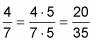##### Algebra I For Dummies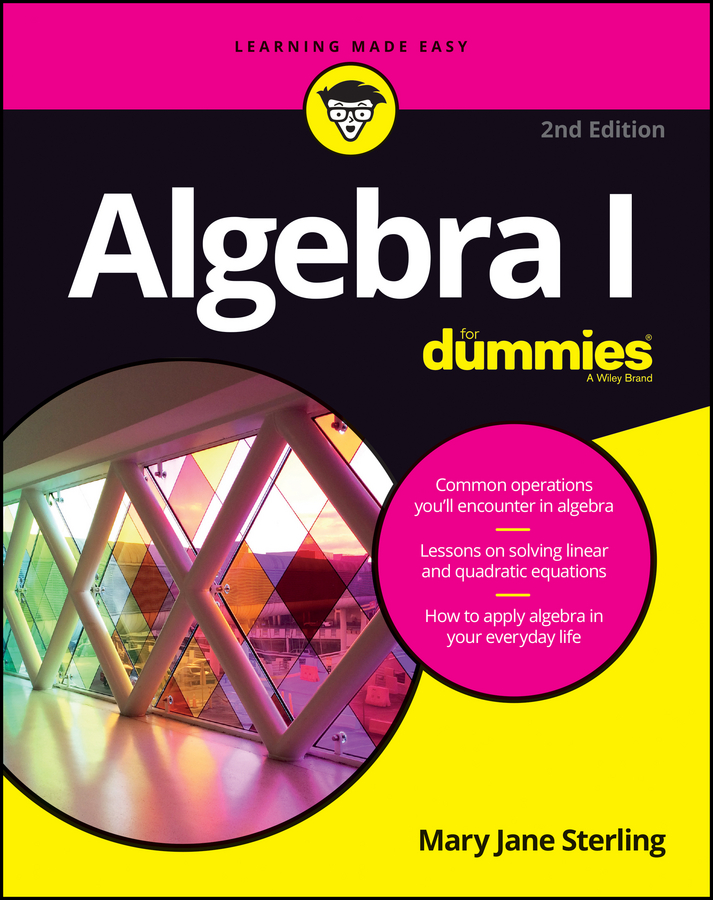Even if fractions look different, they can actually represent the same amount; in other words, one of the fractions will have increased terms compared to the other. You may need to increase the terms of fractions to work with them in an equation.

To increase the terms of a fraction by a certain number, you multiply both the numerator and the denominator by that number. For example, to increase the terms of the fraction 3/4 by 2, multiply both the numerator and the denominator by 2: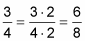Similarly, to increase the terms of the fraction 5/11 by 7, multiply both the numerator and the denominator by 7: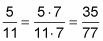Increasing the terms of a fraction doesn’t change its value. Because you’re multiplying the numerator and denominator by the same number, you’re essentially multiplying the fraction by a fraction that equals 1.

One key thing to know is how to increase the terms of a fraction so that the denominator becomes a preset number. Here’s how you do it:

1. Divide the new denominator by the old denominator.

To keep the fractions equal, you have to multiply the numerator and denominator of the old fraction by the same number. This first step tells you what the old denominator was multiplied by to get the new one.

For example, suppose you want to raise the terms of the fraction 4/7 so that the denominator is 35. That is, you’re trying to fill in the question mark here: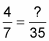Divide 35 by 7, which tells you that the denominator was multiplied by 5.

2. Multiply this result by the old numerator to get the new numerator.

You now know how the two denominators are related. The numerators need to have the same relationship, so multiply the old numerator by the number you found in Step 1.

Multiply 5 by 4, which gives you 20. So here’s the answer: